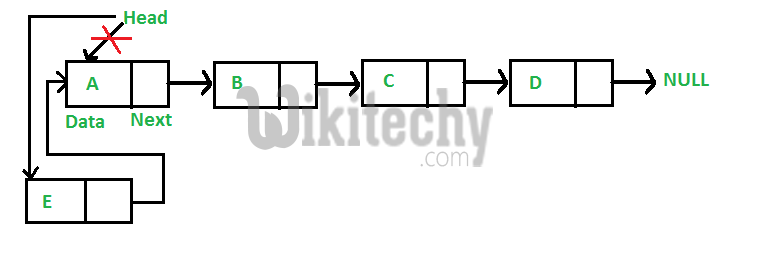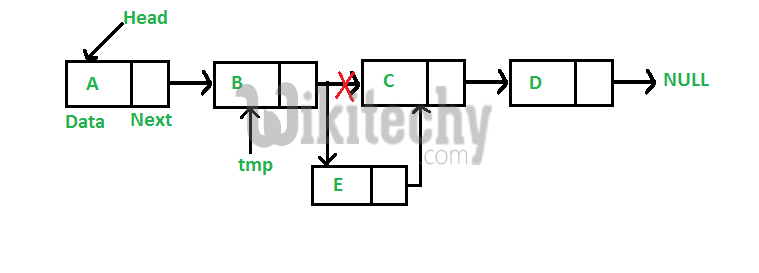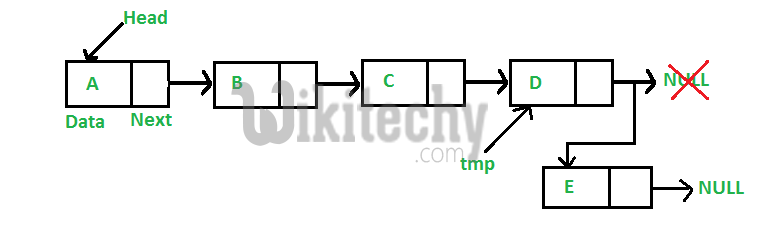# C Programming – Inserting a node in Linked List | Set 2

C Programming -Time complexity of append is O(n) where n is number of nodes in linked list. Since there is a loop from head to end, the function does O(n)

We have introduced Linked Lists in the previous post. We also created a simple linked list with 3 nodes and discussed linked list traversal.

All programs discussed in this post consider following representations of linked list

C Programming:

``````// A linked list node
struct node
{
int data;
struct node *next;
};``````

In this post, methods to insert a new node in linked list are discussed. A node can be added in three ways
1) At the front of the linked list
2) After a given node.
3) At the end of the linked list.

Add a node at the front: (A 4 steps process)
The new node is always added before the head of the given Linked List. And newly added node becomes the new head of the Linked List. For example if the given Linked List is 10->15->20->25 and we add an item 5 at the front, then the Linked List becomes 5->10->15->20->25. Let us call the function that adds at the front of the list is push(). The push() must receive a pointer to the head pointer, because push must change the head pointer to point to the new node (See this)Following are the 4 steps to add node at the front.

C Programming:

``````/* Given a reference (pointer to pointer) to the head of a list
and an int,  inserts a new node on the front of the list. */
void push(struct node** head_ref, int new_data)
{
/* 1. allocate node */
struct node* new_node = (struct node*) malloc(sizeof(struct node));

/* 2. put in the data  */
new_node->data  = new_data;

/* 3. Make next of new node as head */

/* 4. move the head to point to the new node */
}``````

Time complexity of push() is O(1) as it does constant amount of work.

Add a node after a given node: (5 steps process)
We are given pointer to a node, and the new node is inserted after the given node.C Programming:

``````/* Given a node prev_node, insert a new node after the given
prev_node */
void insertAfter(struct node* prev_node, int new_data)
{
/*1. check if the given prev_node is NULL */
if (prev_node == NULL)
{
printf("the given previous node cannot be NULL");
return;
}

/* 2. allocate new node */
struct node* new_node =(struct node*) malloc(sizeof(struct node));

/* 3. put in the data  */
new_node->data  = new_data;

/* 4. Make next of new node as next of prev_node */
new_node->next = prev_node->next;

/* 5. move the next of prev_node as new_node */
prev_node->next = new_node;
}``````

Time complexity of insertAfter() is O(1) as it does constant amount of work.

Add a node at the end: (6 steps process)
The new node is always added after the last node of the given Linked List. For example if the given Linked List is 5->10->15->20->25 and we add an item 30 at the end, then the Linked List becomes 5->10->15->20->25->30.
Since a Linked List is typically represented by the head of it, we have to traverse the list till end and then change the next of last node to new node.Following are the 6 steps to add node at the end

C Programming:

``````/* Given a reference (pointer to pointer) to the head
of a list and an int, appends a new node at the end  */
void append(struct node** head_ref, int new_data)
{
/* 1. allocate node */
struct node* new_node = (struct node*) malloc(sizeof(struct node));

struct node *last = *head_ref;  /* used in step 5*/

/* 2. put in the data  */
new_node->data  = new_data;

/* 3. This new node is going to be the last node, so make next
of it as NULL*/
new_node->next = NULL;

/* 4. If the Linked List is empty, then make the new node as head */
if (*head_ref == NULL)
{
return;
}

/* 5. Else traverse till the last node */
while (last->next != NULL)
last = last->next;

/* 6. Change the next of last node */
last->next = new_node;
return;
}``````

Time complexity of append is O(n) where n is the number of nodes in linked list. Since there is a loop from head to end, the function does O(n) work.
This method can also be optimized to work in O(1) by keeping an extra pointer to tail of linked list

Following is a complete program that uses all of the above methods to create a linked list.

C Programming:

``````// A complete working C program to demonstrate all insertion methods
// on Linked List
#include <stdio.h>
#include <stdlib.h>

// A linked list node
struct node
{
int data;
struct node *next;
};

/* Given a reference (pointer to pointer) to the head of a list and
an int, inserts a new node on the front of the list. */
void push(struct node** head_ref, int new_data)
{
/* 1. allocate node */
struct node* new_node = (struct node*) malloc(sizeof(struct node));

/* 2. put in the data  */
new_node->data  = new_data;

/* 3. Make next of new node as head */

/* 4. move the head to point to the new node */
}

/* Given a node prev_node, insert a new node after the given
prev_node */
void insertAfter(struct node* prev_node, int new_data)
{
/*1. check if the given prev_node is NULL */
if (prev_node == NULL)
{
printf("the given previous node cannot be NULL");
return;
}

/* 2. allocate new node */
struct node* new_node =(struct node*) malloc(sizeof(struct node));

/* 3. put in the data  */
new_node->data  = new_data;

/* 4. Make next of new node as next of prev_node */
new_node->next = prev_node->next;

/* 5. move the next of prev_node as new_node */
prev_node->next = new_node;
}

/* Given a reference (pointer to pointer) to the head
of a list and an int, appends a new node at the end  */
void append(struct node** head_ref, int new_data)
{
/* 1. allocate node */
struct node* new_node = (struct node*) malloc(sizeof(struct node));

struct node *last = *head_ref;  /* used in step 5*/

/* 2. put in the data  */
new_node->data  = new_data;

/* 3. This new node is going to be the last node, so make next of
it as NULL*/
new_node->next = NULL;

/* 4. If the Linked List is empty, then make the new node as head */
if (*head_ref == NULL)
{
return;
}

/* 5. Else traverse till the last node */
while (last->next != NULL)
last = last->next;

/* 6. Change the next of last node */
last->next = new_node;
return;
}

// This function prints contents of linked list starting from head
void printList(struct node *node)
{
while (node != NULL)
{
printf(" %d ", node->data);
node = node->next;
}
}

/* Driver program to test above functions*/
int main()
{
/* Start with the empty list */
struct node* head = NULL;

// Insert 6.  So linked list becomes 6->NULL

// Insert 7 at the beginning. So linked list becomes 7->6->NULL

// Insert 1 at the beginning. So linked list becomes 1->7->6->NULL

// Insert 4 at the end. So linked list becomes 1->7->6->4->NULL

// Insert 8, after 7. So linked list becomes 1->7->8->6->4->NULL

printf("\n Created Linked list is: ");

return 0;
}``````

Output:

` Created Linked list is:  1  7  8  6  4`

#### About the author#### Venkatesan Prabu

Wikitechy Founder, Author, International Speaker, and Job Consultant. My role as the CEO of Wikitechy, I help businesses build their next generation digital platforms and help with their product innovation and growth strategy. I'm a frequent speaker at tech conferences and events.